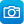# Search results

Year level
Resource type
Learning area

## Refine by topic

Main topic Specific topic Related topic
Listed under:  Dimensions### Measuring our coastline

How long is the Australian coastline? See Dr Derek Muller and Simon Pampena discussing the perimeter of the Australian coastline. Find out how the accuracy of that measurement depends on the length of the 'measuring stick' used. They discuss how a coastline is much like a fractal such as 'Koch's Snowflake'!### Drawing a floor plan

How do we know what a house will look like before it is built? Discover how house plans work by looking at the design of a house that Hugo's family is going to build. See how a floor plan shows the room layout. See drawings of what the house will look like from different views.### Sites2See – measurement for primary

Selected links to a range of interactive and print resources for Measurement topics in K-6 Mathematics.### Working out the areas

Do you know how to work out the area of a square, a rectangle or a triangle? Learn the simple maths formulas needed from this video. What would be the area of a rectangle with a height of 5cm and a length of 3cm?### Using 'Area and perimeter' - Teacher idea

This Teacher idea relates the experiences of teaching R11472 'Area and perimeter - unit of work' both with students in a regular year 3 class and with others online in a virtual classroom. Through digital resources and hands-on tasks the unit explores the early concepts of perimeter and area, including the introduction ...### Turn up the volume - unit of work

In this unit of work students explore and explain the connections between the surface area and volume of different shapes and how each attribute is measured.### TIMES Module 11: Measurement and Geometry: area, volume and surface area - teacher guide

This is a 15-page guide for teachers containing explanations of the derivation of formulas for the areas of parallelograms, trapeziums, rhombuses and kites. Formulas for the volumes and surface areas of prisms and cylinders are obtained. Applications of these formulas are given. A history of the development of these concepts ...### Giant hole: climb through a hole in a sheet of paper

This teaching resource outlines an activity for students to explore the relationship between the area and perimeter of a shape, by using a series of cuts in a piece of paper to create a hole large enough to step through. The resource includes detailed instructions for creating the cuts in the paper, an explanation of how ...### Perimeter and area

This is a six-page HTML resource about solving problems with perimeter and area. It contains one video and nine questions, five of which are interactive. The resource discusses and explains solving problems with area and perimeter to reinforce students' understanding.### Make a cake: measure ingredients

Sarah is following a recipe. There are some different units of measure used in the recipe. Watch the video to see what these measurement words are. How much of each ingredient is needed? How are the ingredients combined to make the cake?### EagleCat: scale it

Explore changes in area when a shape is acted on by a scale factor. Examine changes in volume when an object is acted on by a scale factor. Analyse the changes using whole numbers.### Measures: scaling down

Compare the areas of squares, rectangles and triangles before and after being scaled down (reduced). Notice that 'similar shapes' in the mathematical sense have the same shape but different areas. Explore the relationship between side-length reduction and area reduction when scaling down shapes. This learning object is ...### Measures: volumes

Compare the volumes of a range of rectangular prisms when scaling up (enlarging) side lengths by different ratios. Notice that the rectangular prisms are not similar in the mathematical sense, but it is possible to predict the effect on the volume produced by the scaling of the sides. Identify and describe the relationship ...### Triangle pavers - mathematics activities

This photograph of triangular tiles inlaid in a pavement suggests explorations of the several relationships of these tiles relative to the background square regions. Size relationships and symmetry may be explored. Teachers are encouraged to scan all the ideas suggested here as relevant to the various year level groupings, ...### Glass pyramid - mathematics activities

This is a photograph of a skylight on Parliament House in Canberra. The skylight is in the shape of a square-based pyramid. It suggests investigations involving counting, sequencing, surface area, volume and maxima and minima, as well as connecting pyramids with their historical and cultural place. Determining ways to estimate ...### Area and perimeter - unit of work

The unit allows students to explore the beginning concepts of perimeter and area, including the formal units of cm, m, cm sq, and m sq and to learn the differences between these measurements.### We have it covered - unit of work

This unit allows students to explore the beginning concepts of area, including the formal units of cm, m, cm sq, and m sq.### Living on the edge - unit of work

This unit allows students to explore the beginning concepts of perimeter, including the formal units of cm and m.### Standard yard, Troughton and Simms, c1856

This is a Standard Yard made by Troughton and Simms of London circa 1856. It consists of a bronze bar in a wooden case. The yard is measured between fine lines marked on a gold pin in the well at each end of the bar. It is 113 cm long, 10 cm high and 8 cm wide.### TIMES Module 10: Measurement and Geometry: introduction to measurement - teacher guide

This is a 16-page guide for teachers. It provides an introduction to the initial ideas of measurement, and introduces the measurement of length, area, volume and time.